Vectors and conics

This free course is available to start right now. Review the full course description and key learning outcomes and create an account and enrol if you want a free statement of participation.

Free course

4.2 Circles

Recall that a circle in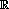2 is the set of points (x, y) that lie at a fixed distance, called the radius, from a fixed point, called the centre of the circle. We can use the techniques of coordinate geometry to find the equation of a circle with a given centre and radius.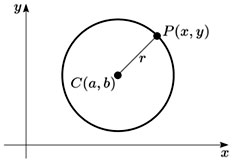Let a circle have centre C(a, b) and radius r. Then, if P(x, y) is an arbitrary point on the circumference of the circle, the distance CP equals r. It follows from the formula for the distance between two points in the plane that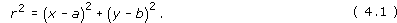Theorem 2

The equation of a circle in2 with centre (a, b) and radius r is

(x − a)2 + (y − b)2 = r2.

For example, it follows from this formula that the circle with centre (−1, 2) and radius √3 has equation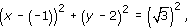which can be simplified to give

x2 + 2x + 1 + y2 − 4y + 4 = 3,

or

x2 + y2 + 2x − 4y + 2 = 0.

Example 51

Determine the equation of each of the following circles, given the centre and radius:

• (a) centre the origin, radius 1;

• (b) centre the origin, radius 4;

• (c) centre (3, 4), radius 2.

We use the standard formula for the equation of a circle of given centre and radius given in Theorem 2.

• (a) This circle has equation

• (x − 0)2 + (y − 0)2 = 12,

• which can be rewritten in the form

• x2 + y2 = 1.

• (b) This circle has equation

• (x − 0)2 + (y − 0)2 = 42,

• which can be rewritten in the form

• x2 + y2 = 16.

• (c) This circle has equation

• (x − 3)2 + (y − 4)2 = 22,

• which can be rewritten in the form

• x2 + y2 − 6x − 8y + 21 = 0.

If we expand the brackets in equation (4.1) and collect the corresponding terms, we can rewrite equation (4.1) in the form

x2 + y2 − 2ax − 2by + (a2 + b2 − r2) = 0.

Then, if we write f for −2a, g for −2b and h for a2 + b2r2, this equation takes the form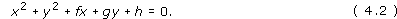Note: The coefficients of x2 and y2 are equal.

In many situations, equation (4.1) is more useful than equation (4.2) for determining the equation of a particular circle.

We have seen that the equation of a circle can be written in the formOn the other hand, given an equation of the form (4.2), can we determine whether it represents a circle? If it does represent a circle, can we determine its centre and radius?

For example, consider the set of points (x, y) in the plane that satisfy the equation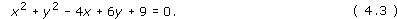Note: In equation (4.3) the coefficients of x2 and y2 are both 1.

In order to transform equation (4.3) into an equation of the form equation (4.1), we use a technique called ‘completing the square’. We rewrite the terms that involve only x and the terms that involve only y, as follows: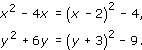Note: −2 is half the coefficient of x and +3 is half the coefficient of y in equation (4.3).

Substituting the expressions above into equation (4.3), we obtain

((x − 2)2 − 4) + ((y + 3)2 − 9) + 9 = 0,

that is,

(x − 2)2 + (y + 3)2 = 4.

Note: We can ‘read off’ the centre and radius of the circle from this equation.

It follows that equation (4.3) represents a circle with centre (2, −3) and radius 2.

In general, we can use the same method, ‘completing the square’, to rewrite the equation

x2 + y2 + fx + gy + h = 0

in the form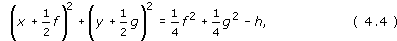Note: Again, the coefficients of x2 and y2 are both 1.

The constant in the first bracket is half the coefficient of x in the previous equation, and the constant in the second bracket is half the coefficient of y.

Theorem 3

An equation of the form

x2 + y2 + fx + gy + h = 0

represents a circle with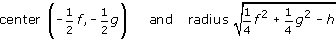if and only if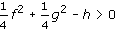.

Remark: It follows from equation (4.4) that if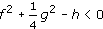, then there are no points (x, y) that satisfy the equation x2 + y2 + fx + gy + h = 0; and if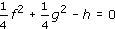, then the given equation simply represents the single point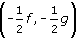.

Example 52

Determine the condition on the numbers f, g and h in the equation

x2 + y2 + fx + gy + h = 0

for the circle with this equation to pass through the origin.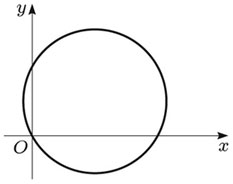Since the origin lies on the circle, its coordinates (0, 0) must satisfy the equation of the circle. Thus

02 + 02 + f × 0 + g × 0 + h = 0,

which reduces to the condition h = 0.

Example 53

Determine the centre and radius of each of the circles given by the following equations.

• (a) x2 + y2 − 2x − 6y + 1 = 0

• (b) 3x2 + 3y2 − 12x − 48y = 0

• (a) We can complete the square in the equation

• x2 + y2 − 2x − 6y + 1 = 0

• to obtain

• (x − 1)2 − 1 + (y − 3)2 − 9 + 1 = 0,

• or

• (x − 1)2 + (y − 3)2 = 9.

• So the circle has centre (1, 3) and radius √9 = 3.

• (Alternatively, use the general formula for centre and radius in Theorem 3 with f = −2, g = −6 and h = 1.)

• (b) Here the coefficients of x2 and y2 are both 3, so we divide the equation by 3 to obtain

•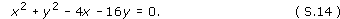• Completing the square gives

• (x − 2)2 − 4 + (y − 8)2 − 64 = 0,

• or

• (x − 2)2 + (y − 8)2 + 68.

• So the circle has centre (2, 8) and radius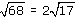• (Alternatively, apply the general formula for centre and radius to the equation in the form of equation (S.14), with f = −4, g = −16 and h = 0.)

Example 54

Determine the set of points (x, y) in2 that satisfy each of the following equations.

• (a) x2 + y2 + x + y + 1 = 0

• (b) x2 + y2 − 2x + 4y + 5 = 0

• (c) 2x2 + 2y2 + x − 3y − 5 = 0

• (a) If we complete the square in the equation

•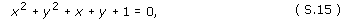• we obtain the equation

•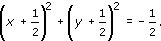• This equation represents the empty set, since its left-hand side is always non-negative whereas its right-hand side is negative.

• (Alternatively, we can use information about the quantity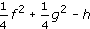. In equation (S.15), we have f = 1, g = 1 and h = 1. Thus

•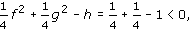• so the set must be empty.)

• (b) If we complete the square in the equation

•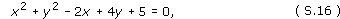• we obtain the equation

• (x − 1)2 + (y + 2)2 = 0.

• Thus the set in the plane represented by equation (S.16) is the single point (1, −2).

• (Alternatively, we can use information about the quantity. In equation (S.16), we have f = −2, g = 4 and h = 5. Thus

•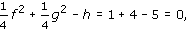• so the set is the single point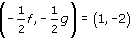• (c) Here the coefficients of x2 and y2 are both 2, so we divide the equation by 2 to give

•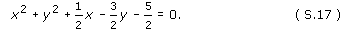• If we complete the square in equation (S.17), we obtain the equation

•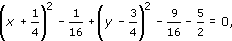• that is,

•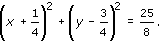• Thus the set in the plane represented by equation (S.17) is a circle with centre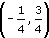and radius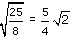.

• (Alternatively, we can use information about the quantity. In equation (S.17), we have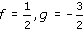and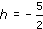. Thus

•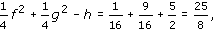• so the set is the circle with centreand radiusM208_1